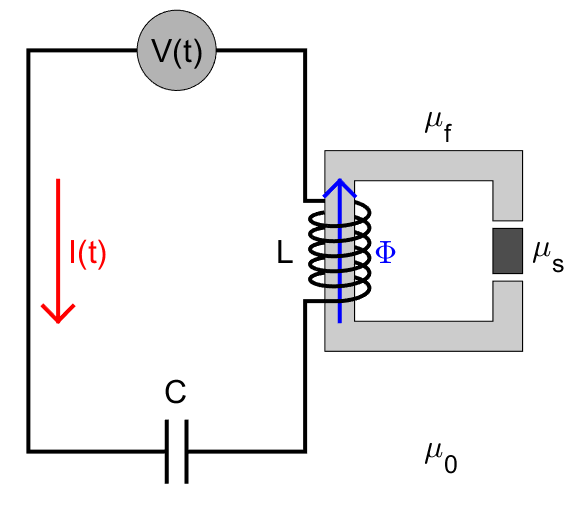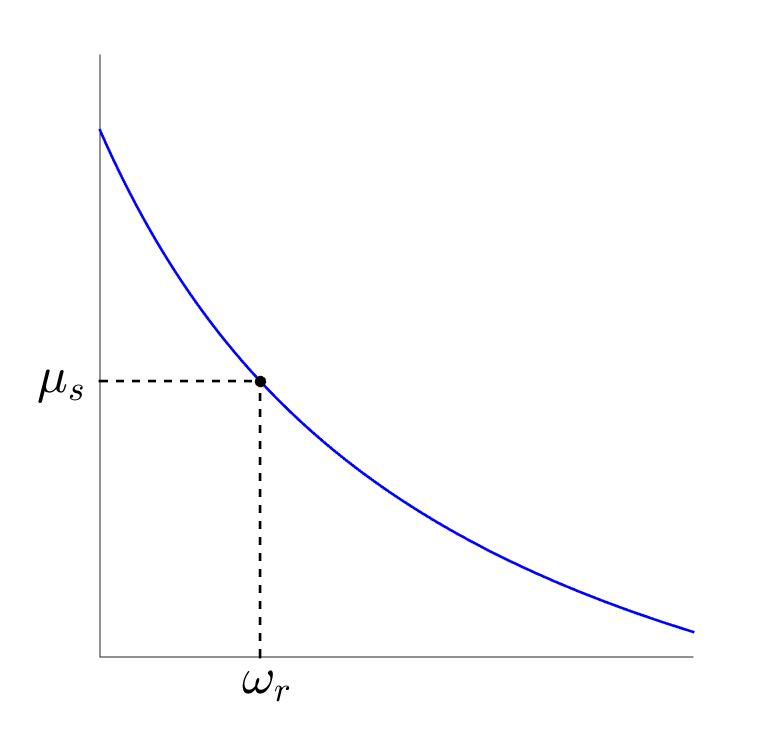# Laboratory Measurements

Magnetic permeability measurements are an integral part of classifying physical rock properties. Here, we present the general approach for measuring a rock’s magnetic permeability. Background theory is then provided for a simplified instrument configuration.

## General ApproachFig. 27 Electrical circuit diagram representing a simplified instrument configuration. The inductive element $$L$$ depends on the magnetic permeability of the sample $$\mu_s$$.

Magnetic permeability measurements can be understood by considering the forced, in-series LC circuit diagram in Fig. 27. The current $$I(t)$$ within the wire is generated by a driving voltage $$V(t)$$. This current is defined by the following ordinary differential equation:

(12)$\frac{d^2 I (t)}{d t} + \frac{1}{LC} I(t) = F(t)$

For our circuit, the inductive element $$L$$ depends on the magnetic permeability $$\mu_s$$ of the rock sample. This relationship is discussed more in the following section. $$C$$ represents a known capacitive element, and $$F(t)$$ is used to represent the driving voltage. From Eq. (12), the circuit has a natural resonance frequency $$\omega_r$$ at:Fig. 28 Representation of an experimentally derived curve, for a particular instrument, which characterizes an empirical relationship between $$\mu_s$$ and $$\omega_r$$.
(13)$\omega_r = \frac{1}{\sqrt{LC}}$

Since $$C$$ is known, Eq. (13) implies there exists an explicit relationship between $$\omega_r$$ and $$\mu_s$$. Unfortunately, this relationship is not particularly straightforward, and depends on the configuration of the instrument. In most cases, an experimentally derived curve is used to characterize an empirical relationship between $$\omega_r$$ and $$\mu_s$$. Once established, the magnetic permeability of a sample may be obtained by 1) determining the resonance frequency of the circuit, then 2) finding the corresponding magnetic permeability value on the curve ([CE91]). This is illustrated in Fig. 28.

## Background Theory: A Simplified Experiment

Once again, let us consider our in-series LC circuit. For sensitive permeability measurements, a portion of the wire is frequently wound around a ferrite. Ferrites are non-conductive and extremely permeable ($$\mu_{f} \sim 1000\mu_0$$). As a result, they become extremely magnetized when exposed to a magnetic field, but experience negligible EM induction under 5 kHz ([CE91]). The ferrite does not form a closed path. Within the gap, a rock of magnetic permeability $$\mu_s$$ is placed. The coil-ferrite system acts as an inductive element for the circuit, and is denoted by $$L$$. Thus, the current within the wire can be describe using Eq. (12).

The driving voltage $$V(t)$$ generates a current $$I(t)$$ within the wire. This current creates a magnetic field $$H$$ within the coil. If the material within the coil was conductive, it would experience an electromotive force. Because ferrites are purely magnetic, they experience a magnetomotive force $$\mathcal{F}$$ instead. Whereas electromotive forces oppose the magnetic field, magnetomotive forces re-enforce it. By neglecting edge effects near the ends of the coil, the magnetomotive force experienced by the ferrite is:

(14)$\mathcal{F} = NI = H \Delta S$

where $$N$$ is the number of turns the coil has, and $$\Delta S$$ is the length of the coil. Because ferrites are so permeable, they behave like a magnetic circuit in this case. The applied magnetomotive force generates a magnetic flux $$\Phi$$, which permeates through the material. This can be described using Hopkinson’s law , which is analogous to Ohm’s law:

(15)$\mathcal{F} = \Phi \Re$

where $$\Phi$$ is the magnetic flux along the path of the ferrite, and $$\Re$$ is defined as the magnetic reluctance. Magnetic reluctance represents the ratio of magnetomotive force to induced magnetic flux. If our ferrite forms a closed path, has uniform cross-sectional area $$A$$, and total length $$\ell$$, its magnetic reluctance is given by:

(16)$\Re = \frac{\ell}{\mu_f A}$

In our experiment however, there is a gap containing a rock sample. Introduction of a sample changes the magnetic reluctance of the circuit ([CE91]). Like electrically resistive elements, magnetically reluctant elements may be added in series. If the cross-sectional area remains constant:

(17)$\Re = \sum_k \frac{\ell_k}{\mu_k A}$

Eq. (17) can therefore be used to describe the magnetic reluctance of our system in the absence of a rock sample. When a rock sample is placed within the gap, it affects the magnetic reluctance. In most laboratory experiments, the magnetic reluctance is given by ([CE91]):

(18)$\Re = \Re_0 + \frac{\alpha}{\mu_s}$

where $$\Re_0$$ and $$\alpha$$ can be experimentally determined, and depend on the geometry of the instrument. By definition of the self-inductance, and by using Eqs. (15) and (18):

(19)$L = \frac{N \Phi}{I} = \frac{N \mathcal{F}}{I \Re} = \frac{N^2}{\Re}$

Therefore, the self-inductance of the circuit is inversely proportional to the magnetic reluctance. Using Eqs. (13), (18) and (19), the magnetic permeability of a rock sample may be determined by the following expression:

(20)$\mu_s = \frac{\alpha}{C (N \omega_r )^2 - \Re_0}$